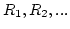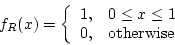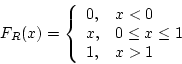Next: Inverse Transform Technique Up: Simulation CSCI 6337 Previous: Poker Test

# Random Variate Generation

Now that we have learned how to generate a uniformly distributed random variable, we will study how to produce random variables of other distribution using the uniformly distributed random variable.

The techniques discussed include inverse transform and convolution. Also discussed is the acceptance-rejection technique.

All work here assume the existence of a source of uniform (0,1) random numbers,• pdf• cdfSubsections

Meng Xiannong 2002-10-18